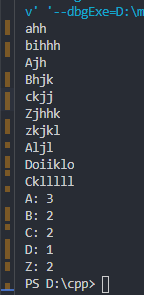2021-11-26 00:48

# 求解c语言统计不同字母开头人数

• 好问题 提建议
• 收藏

#### 1条回答默认 最新

•已采纳

你题目的解答代码如下：

``````#include<stdio.h>
int main()
{
char a = {};
int n=10,i;
int c = {0};
for (i = 0; i < n; i++)
{
scanf("%s", &a[i]);
}
for (i = 0; i < n; i++)
{
if ((a[i] >= 'A') && (a[i] <= 'Z')) {
c[a[i]-'A']++;
}
else if ((a[i] >= 'a') && (a[i] <= 'z')) {
c[a[i]-'a']++;
}
}
for (i = 0; i < 26; i++)
{
if (c[i]>0)
printf("%c: %d\n", i+'A', c[i]);
}
return 0;
}
``````如有帮助，望采纳！谢谢!

编辑记录

已采纳该答案
评论
解决 无用
打赏 举报